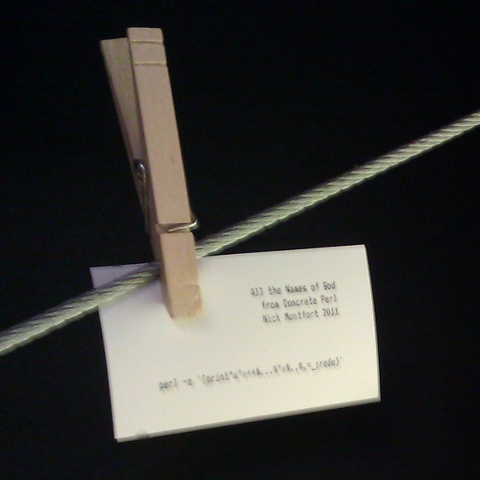# Concrete Perl

h            d d     k x  v  d r k y  p  s b a  b  a  n  i  k  d   u  u
v   r  c q  i  e  z   j s s  v h   t l  i  r k k n  k       n n     m
z b    q   b   k x  m  d u  z f  s  g p u z v y       v m  f   s
i  u  p  p z   r n t  k f   b h v  q l  x w h x  f  x    c i w     v f
k h   l  a i      o q  s z n z  u n c l    w      d     a  d a  m j  b e
m  n b q o u o e  n   s    r b o j     b  q q t q s   f n i  f     u  l

### Concrete Perl

#### a set of four concrete poems realized as 32-character Perl programs

##### by Nick Montfort

You can download the linked Perl files and/or simply copy and paste the following four lines, which correspond to the four titles above:

`perl -e '{print"a"x++\$...\$"x\$.,\$,=_;redo}'`
`perl -e '{print\$,=\$"x(\$.+=.01),a..z;redo}'`
`perl -e '{print" ".chr for 32..126;redo}'`
`perl -e '{print\$",\$_=(a..z)[rand\$=];redo}'`

For purposes of determining the platform precisely and counting characters, the rules of Perl Golf are
used. These rules, for instance, do not count the (optional) newline at the end of a one-line program. The Concrete Perl programs work on all standard versions of Perl 5.8.0 and have been verified as 32 characters long using a count program.

These programs are also written to work and to be visually pleasing on terminal windows (or terminals) of any geometry.

To present them all at once, you can tile four windows and run one program in each window. For instance, running Linux with Compiz as the window manager and the Grid plugin installed and active, create four windows and assign them to the four corners of the screen using Ctrl-Alt-Num Pad 7, Ctrl-Alt-Num Pad 9, Ctrl-Alt-Num Pad 1, and Ctrl-Alt-Num Pad 3. In this
mode if the resolution of the display is not particularly high, you may wish to decrease the font size a notch in each window.

Concrete Perl was released onto the demoscene and discussed by me for the first time in my talk “Beyond Data-Driven Poetry: ppg256 and Concrete Perl,” on the panel “Literary Practice and the Digital Humanities, Redux: Data as/and Poetry,” Digital Humanities 2011, Stanford, June 22, 2011.

I have printed the four programs/poems on a dot matrix printer on business cards and am handing them out and, in some cases, adding them to the “Interactive Poetry Wall” at Stanford University’s “Coho” coffeehouse.p  j  o c v v     n  v  t  g k   t i      s h    f j   v  e d    v c e  b
p z          p  e     s s d    o f z   v  p s  t  z   i b  f j w     l
p  z y      f j  w k k p k y n  v f g u m r m k x i  w  l s a t n b a f
w   q    y u t p r p w     p       m d   x j c j   j n z k a   j  z  i s
w a    w q k       j   y y   k c r    d i         b p  f   z h  i x    i
c  o  x  f  p f     u d   g z y f b y  v     q    v  g v    o      j l